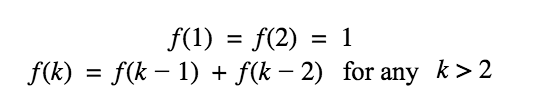# Fibonacci Sequence

Time Limit: 1 Second    Memory Limit: 65536 KB

Your job is to take an integer x as the input and compute f(x) mod 109, where f(x) is the x-th value in the well-known Fibonacci sequence.

Note that Fibonacci sequence is defined as follows:## Input

The first line of the input includes the number of test cases, 1≤t≤1000. Each test case is a line containing an integer 1≤x≤248.

## Output

For each test case, print one line containing f(x) mod 109.

## Sample Input

```11
1
2
8
20
46
60
3749999998
3749999999
3750000000
3750000001
281474976710656 ```

## Sample Output

```1
1
21
6765
836311903
8755920
499999999
500000001
0
500000001
309764667
```
Submit

Source: 13th Iran Nationwide Internet Contest - Final